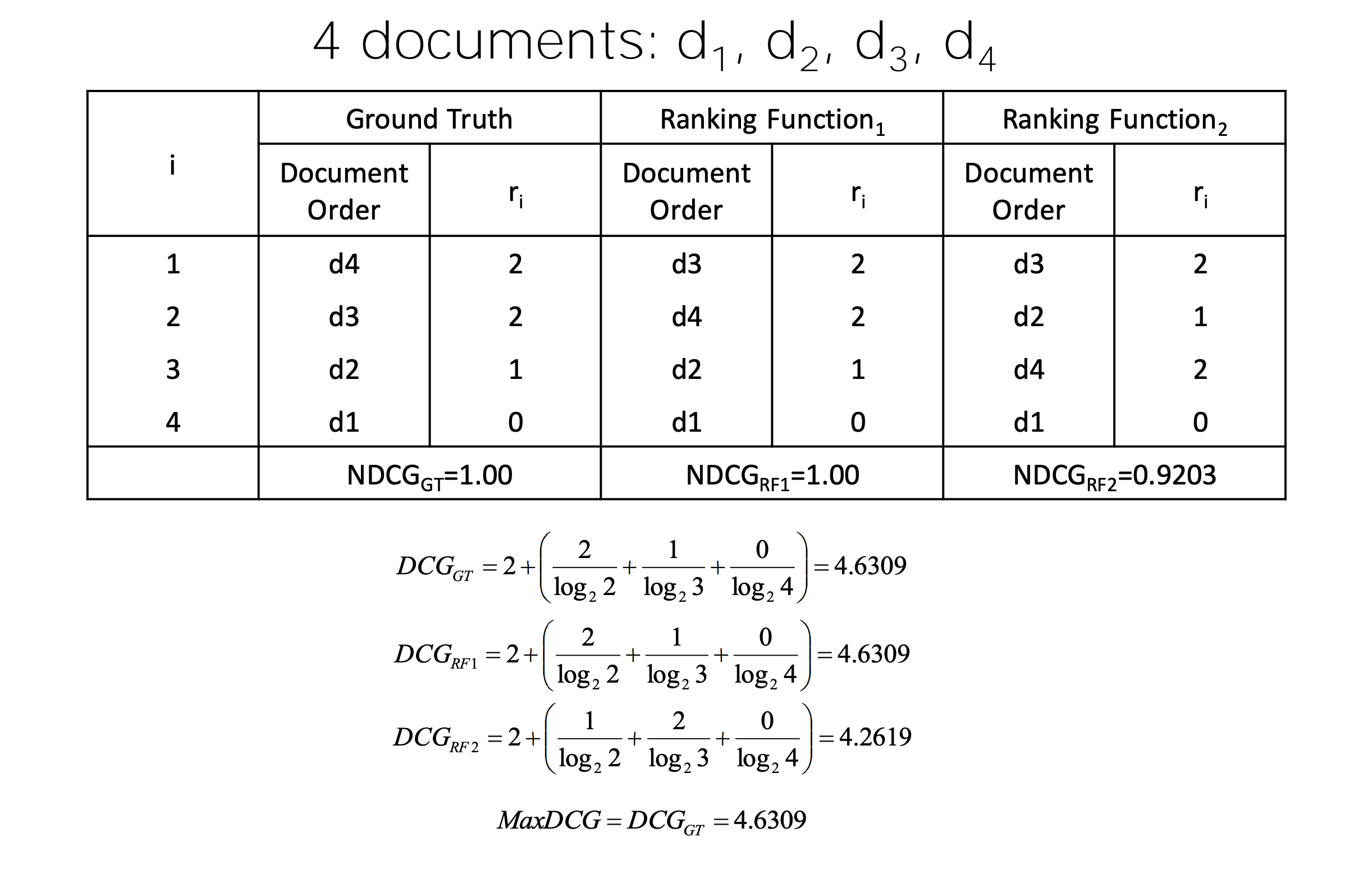# “Common information retrieval evaluation metrics”

## Rank-Based Measures:

1. Binary relevance
• Precision@K (P@K)
• Mean Average Precision (MAP)
• Mean Reciprocal Rank (MRR)
2. Multiple levels of relevance
• Normalized Discounted Cumulative Gain (NDCG)

## Precision@K

1. Set a rank threshold K
2. Compute % relevant in top K
3. Ignores documents ranked lower than K
4. Example:
• Prec@3 of 2/3
• Prec@4 of 2/4
• Prec@5 of 3/5## Mean Average Precision

1. Consider rank position of each relevant doc $K_{1}$, $K_{2}$,…, $K_{R}$.
2. Compute Precision@K for each $K_{1}$, $K_{2}$,…, $K_{R}$
3. Average precision = average of P@K
4. Example: $\frac{1}{3} \cdot\left(\frac{1}{1}+\frac{2}{3}+\frac{3}{5}\right) \approx 0.76$1. MAP is Average Precision across multiple queries/rankings
2. MAP is macro-averaging: each query counts equally

## When There’s only 1 Relevant Document

1. Scenarios:
• known-item search
• looking for a fact
2. Search Length = Rank of the answer
• measures a user’s effort

## Mean Reciprocal Rank

1. Consider rank position, K, of first relevant doc
2. Reciprocal Rank score $=\frac{1}{K}$
3. MRR is the mean RR across multiple queries

## Discounted Cumulative Gain

1. Popular measure for evaluating web search and related tasks
2. Two assumptions:
• Highly relevant documents are more useful than marginally relevant document
• the lower the ranked position of a relevant document, the less useful it is for the user, since it is less likely to be examined
3. Uses graded relevance as a measure of usefulness, or gain, from examining a document
4. Gain is accumulated starting at the top of the ranking and may be reduced, or discounted, at lower ranks
5. Typical discount is 1/log (rank)
6. DCG is the total gain accumulated at a particular rank p: $DCG_{p}=r e l_{1}+\sum_{i=2}^{p} \frac{r e l_{i}}{\log _{2} i}$

## DCG Examples:

1. 10 ranked documents judged on 0-3 relevance scale: 3, 2, 3, 0, 0, 1, 2, 2, 3, 0
2. discounted gain: 3, 2/1, 3/1.59, 0, 0, 1/2.59, 2/2.81, 2/3, 3/3.17, 0 = 3, 2, 1.89, 0, 0, 0.39, 0.71, 0.67, 0.95, 0
3. DCG: 3, 5, 6.89, 6.89, 6.89, 7.28, 7.99, 8.66, 9.61, 9.61

## NDCG:

1. Normalized Cumulative Gain (NDCG) at rank n
• Normalize DCG at rank n by the DCG value at rank n of the ideal ranking
• The ideal ranking would first return the documents with the highest relevance level, then the next highest relevance level, etc
• Compute the precision (at rank) where each (new) relevant document is retrieved => p(1),…,p(k), if we have k rel.docs
2. An Example:## YONG HUANG

Hi, I'm Yong Huang. I am a Ph.D. student in Computer Science at UC Irvine, I am interested in machine learning for healthcare, before coming to UC Irvine, I did my Master at Cornell Tech and did my undergrad at Tongji University. Thank you for visiting my site. I recently removed several blogs because I found mathjax is broken, sorry about the incovenience.